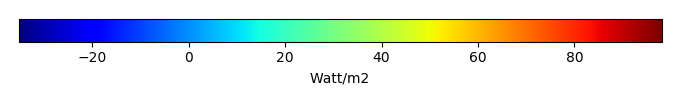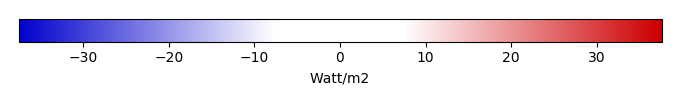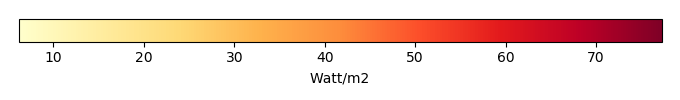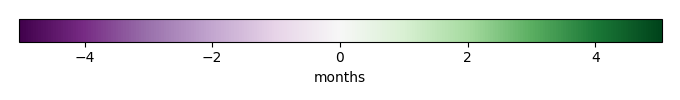# Mean State

Period Mean (original grids) [Watt/m2]
Model Period Mean (intersection) [Watt/m2]
Model Period Mean (complement) [Watt/m2]
Benchmark Period Mean (intersection) [Watt/m2]
Benchmark Period Mean (complement) [Watt/m2]
Bias [Watt/m2]
RMSE [Watt/m2]
Phase Shift [months]
Bias Score 
RMSE Score 
Seasonal Cycle Score 
Spatial Distribution Score 
Overall Score 
Benchmark [-] 44.1
CRUNCEPv7 [-] 52.3 51.6 63.7 44.2 12.4 7.78 20.8 1.29 0.38 0.22 0.81 0.72 0.47
GSWP3v1 [-] 43.5 43.0 49.6 44.2 12.4 -0.924 16.0 1.50 0.39 0.27 0.76 0.89 0.51
WFDEI [-] 54.0 53.4 60.8 44.2 12.4 9.33 16.0 1.09 0.42 0.27 0.84 0.89 0.54
Period Mean (original grids) [Watt/m2]
Model Period Mean (intersection) [Watt/m2]
Model Period Mean (complement) [Watt/m2]
Benchmark Period Mean (intersection) [Watt/m2]
Benchmark Period Mean (complement) [Watt/m2]
Bias [Watt/m2]
RMSE [Watt/m2]
Phase Shift [months]
Bias Score 
RMSE Score 
Seasonal Cycle Score 
Spatial Distribution Score 
Overall Score 
Benchmark [-] 57.5
CRUNCEPv7 [-] 60.0 60.1 87.5 57.5 20.8 2.66 21.1 1.02 0.52 0.38 0.89 0.96 0.63
GSWP3v1 [-] 49.9 50.0 65.5 57.5 20.8 -7.52 20.5 0.609 0.43 0.43 0.96 0.92 0.63
WFDEI [-] 62.9 63.2 78.8 57.5 20.8 5.74 16.9 0.928 0.65 0.43 0.91 0.98 0.68
Period Mean (original grids) [Watt/m2]
Model Period Mean (intersection) [Watt/m2]
Model Period Mean (complement) [Watt/m2]
Benchmark Period Mean (intersection) [Watt/m2]
Benchmark Period Mean (complement) [Watt/m2]
Bias [Watt/m2]
RMSE [Watt/m2]
Phase Shift [months]
Bias Score 
RMSE Score 
Seasonal Cycle Score 
Spatial Distribution Score 
Overall Score 
Benchmark [-] 52.8
CRUNCEPv7 [-] 60.9 60.7 69.0 53.0 19.6 8.02 21.3 0.435 0.50 0.44 0.96 0.89 0.65
GSWP3v1 [-] 55.1 54.9 61.8 53.0 19.6 2.23 18.9 0.441 0.55 0.48 0.96 0.93 0.68
WFDEI [-] 63.8 63.9 64.7 53.0 19.6 11.0 19.3 0.496 0.54 0.47 0.95 0.93 0.67
Period Mean (original grids) [Watt/m2]
Model Period Mean (intersection) [Watt/m2]
Model Period Mean (complement) [Watt/m2]
Benchmark Period Mean (intersection) [Watt/m2]
Benchmark Period Mean (complement) [Watt/m2]
Bias [Watt/m2]
RMSE [Watt/m2]
Phase Shift [months]
Bias Score 
RMSE Score 
Seasonal Cycle Score 
Spatial Distribution Score 
Overall Score 
Benchmark [-] 18.0
CRUNCEPv7 [-] -1.20 -0.937 -5.58 18.1 5.64 -19.3 26.5 0.628 0.46 0.50 0.95 0.47 0.58
GSWP3v1 [-] 14.5 14.9 6.76 18.1 5.64 -3.68 20.8 0.596 0.79 0.48 0.95 0.94 0.73
WFDEI [-] -5.83 -5.52 -11.1 18.1 5.64 -23.9 29.6 0.793 0.39 0.51 0.94 0.28 0.53
Period Mean (original grids) [Watt/m2]
Model Period Mean (intersection) [Watt/m2]
Model Period Mean (complement) [Watt/m2]
Benchmark Period Mean (intersection) [Watt/m2]
Benchmark Period Mean (complement) [Watt/m2]
Bias [Watt/m2]
RMSE [Watt/m2]
Phase Shift [months]
Bias Score 
RMSE Score 
Seasonal Cycle Score 
Spatial Distribution Score 
Overall Score 
Benchmark [-] 22.1
CRUNCEPv7 [-] 28.9 27.5 35.7 22.8 10.8 7.44 20.5 1.79 0.17 0.16 0.73 0.48 0.34
GSWP3v1 [-] 26.9 25.6 32.5 22.8 10.8 5.08 15.0 1.34 0.27 0.23 0.80 0.80 0.47
WFDEI [-] 38.3 37.7 41.0 22.8 10.8 16.0 18.6 1.32 0.17 0.21 0.81 0.95 0.47
Period Mean (original grids) [Watt/m2]
Model Period Mean (intersection) [Watt/m2]
Model Period Mean (complement) [Watt/m2]
Benchmark Period Mean (intersection) [Watt/m2]
Benchmark Period Mean (complement) [Watt/m2]
Bias [Watt/m2]
RMSE [Watt/m2]
Phase Shift [months]
Bias Score 
RMSE Score 
Seasonal Cycle Score 
Spatial Distribution Score 
Overall Score 
Benchmark [-] 27.0
CRUNCEPv7 [-] 12.9 12.9 17.0 27.1 8.81 -14.1 22.5 1.28 0.57 0.53 0.86 0.89 0.68
GSWP3v1 [-] 22.9 22.9 27.6 27.1 8.81 -4.02 15.1 0.642 0.77 0.60 0.93 0.88 0.76
WFDEI [-] 6.76 6.77 13.3 27.1 8.81 -20.2 25.1 1.04 0.47 0.59 0.90 0.71 0.65
Period Mean (original grids) [Watt/m2]
Model Period Mean (intersection) [Watt/m2]
Model Period Mean (complement) [Watt/m2]
Benchmark Period Mean (intersection) [Watt/m2]
Benchmark Period Mean (complement) [Watt/m2]
Bias [Watt/m2]
RMSE [Watt/m2]
Phase Shift [months]
Bias Score 
RMSE Score 
Seasonal Cycle Score 
Spatial Distribution Score 
Overall Score 
Benchmark [-] 28.4
CRUNCEPv7 [-] 37.0 36.6 42.2 28.4 24.4 8.08 20.9 0.919 0.66 0.48 0.90 0.92 0.69
GSWP3v1 [-] 36.4 36.2 40.6 28.4 24.4 7.78 18.9 0.650 0.66 0.54 0.94 0.94 0.72
WFDEI [-] 35.8 35.1 44.0 28.4 24.4 6.50 21.8 0.813 0.61 0.50 0.92 0.79 0.66
Period Mean (original grids) [Watt/m2]
Model Period Mean (intersection) [Watt/m2]
Model Period Mean (complement) [Watt/m2]
Benchmark Period Mean (intersection) [Watt/m2]
Benchmark Period Mean (complement) [Watt/m2]
Bias [Watt/m2]
RMSE [Watt/m2]
Phase Shift [months]
Bias Score 
RMSE Score 
Seasonal Cycle Score 
Spatial Distribution Score 
Overall Score 
Benchmark [-] 40.3
CRUNCEPv7 [-] 53.8 53.9 56.3 40.6 11.0 12.6 23.4 0.392 0.50 0.44 0.97 0.90 0.65
GSWP3v1 [-] 37.8 37.4 41.2 40.6 11.0 -3.37 19.3 0.488 0.52 0.50 0.96 0.94 0.68
WFDEI [-] 48.5 48.4 50.9 40.6 11.0 7.43 18.9 0.439 0.64 0.47 0.97 0.95 0.70
Period Mean (original grids) [Watt/m2]
Model Period Mean (intersection) [Watt/m2]
Model Period Mean (complement) [Watt/m2]
Benchmark Period Mean (intersection) [Watt/m2]
Benchmark Period Mean (complement) [Watt/m2]
Bias [Watt/m2]
RMSE [Watt/m2]
Phase Shift [months]
Bias Score 
RMSE Score 
Seasonal Cycle Score 
Spatial Distribution Score 
Overall Score 
Benchmark [-] 15.2
CRUNCEPv7 [-] -9.23 -8.80 -15.9 15.2 3.78 -24.2 30.4 1.07 0.38 0.49 0.91 0.22 0.50
GSWP3v1 [-] 3.51 3.92 -2.55 15.2 3.78 -11.4 24.3 1.14 0.63 0.45 0.90 0.80 0.65
WFDEI [-] -15.5 -15.1 -21.6 15.2 3.78 -30.5 35.7 1.19 0.30 0.49 0.90 0.16 0.47
Period Mean (original grids) [Watt/m2]
Model Period Mean (intersection) [Watt/m2]
Model Period Mean (complement) [Watt/m2]
Benchmark Period Mean (intersection) [Watt/m2]
Benchmark Period Mean (complement) [Watt/m2]
Bias [Watt/m2]
RMSE [Watt/m2]
Phase Shift [months]
Bias Score 
RMSE Score 
Seasonal Cycle Score 
Spatial Distribution Score 
Overall Score 
Benchmark [-] 56.5
CRUNCEPv7 [-] 66.7 67.3 54.7 56.9 15.0 9.87 17.9 0.507 0.68 0.59 0.96 0.97 0.76
GSWP3v1 [-] 61.4 61.8 53.7 56.9 15.0 4.50 14.8 0.427 0.72 0.65 0.97 0.88 0.77
WFDEI [-] 68.9 69.8 51.9 56.9 15.0 12.1 18.9 0.560 0.63 0.59 0.96 0.98 0.75
Period Mean (original grids) [Watt/m2]
Model Period Mean (intersection) [Watt/m2]
Model Period Mean (complement) [Watt/m2]
Benchmark Period Mean (intersection) [Watt/m2]
Benchmark Period Mean (complement) [Watt/m2]
Bias [Watt/m2]
RMSE [Watt/m2]
Phase Shift [months]
Bias Score 
RMSE Score 
Seasonal Cycle Score 
Spatial Distribution Score 
Overall Score 
Benchmark [-] 20.2
CRUNCEPv7 [-] 23.8 23.6 29.1 20.4 7.08 3.85 21.7 0.553 0.67 0.49 0.93 0.62 0.64
GSWP3v1 [-] 20.3 19.9 30.3 20.4 7.08 0.611 15.7 0.772 0.71 0.62 0.92 0.80 0.73
WFDEI [-] 14.8 14.5 22.9 20.4 7.08 -4.98 20.0 0.552 0.62 0.56 0.95 0.55 0.65
Period Mean (original grids) [Watt/m2]
Model Period Mean (intersection) [Watt/m2]
Model Period Mean (complement) [Watt/m2]
Benchmark Period Mean (intersection) [Watt/m2]
Benchmark Period Mean (complement) [Watt/m2]
Bias [Watt/m2]
RMSE [Watt/m2]
Phase Shift [months]
Bias Score 
RMSE Score 
Seasonal Cycle Score 
Spatial Distribution Score 
Overall Score 
Benchmark [-] 34.3
CRUNCEPv7 [-] 33.4 38.7 11.4 34.6 11.5 4.09 22.9 0.917 0.55 0.46 0.91 0.78 0.63
GSWP3v1 [-] 31.1 36.9 6.93 34.6 11.5 2.33 19.5 0.795 0.59 0.50 0.92 0.92 0.68
WFDEI [-] 32.2 38.0 8.37 34.6 11.5 3.20 22.7 0.854 0.55 0.48 0.92 0.70 0.63
Period Mean (original grids) [Watt/m2]
Model Period Mean (intersection) [Watt/m2]
Model Period Mean (complement) [Watt/m2]
Benchmark Period Mean (intersection) [Watt/m2]
Benchmark Period Mean (complement) [Watt/m2]
Bias [Watt/m2]
RMSE [Watt/m2]
Phase Shift [months]
Bias Score 
RMSE Score 
Seasonal Cycle Score 
Spatial Distribution Score 
Overall Score 
Benchmark [-] 22.7
CRUNCEPv7 [-] 57.0 59.6 54.7 22.8 18.3 37.5 44.0 1.17 0.50 0.50 0.95 0.80 0.65
GSWP3v1 [-] 46.1 49.3 43.1 22.8 18.3 27.3 36.5 1.21 0.51 0.51 0.94 0.79 0.65
WFDEI [-] 55.1 57.5 52.9 22.8 18.3 35.3 41.7 1.19 0.52 0.51 0.94 0.74 0.65
Period Mean (original grids) [Watt/m2]
Model Period Mean (intersection) [Watt/m2]
Model Period Mean (complement) [Watt/m2]
Benchmark Period Mean (intersection) [Watt/m2]
Benchmark Period Mean (complement) [Watt/m2]
Bias [Watt/m2]
RMSE [Watt/m2]
Phase Shift [months]
Bias Score 
RMSE Score 
Seasonal Cycle Score 
Spatial Distribution Score 
Overall Score 
Benchmark [-] 17.0
CRUNCEPv7 [-] -1.15 -0.566 -10.7 17.0 4.51 -18.0 26.8 1.16 0.51 0.49 0.88 0.50 0.57
GSWP3v1 [-] 9.28 9.85 0.800 17.0 4.51 -7.62 22.5 1.05 0.71 0.47 0.90 0.88 0.69
WFDEI [-] -8.84 -8.36 -16.0 17.0 4.51 -25.7 31.8 1.14 0.39 0.51 0.88 0.24 0.50
Period Mean (original grids) [Watt/m2]
Model Period Mean (intersection) [Watt/m2]
Model Period Mean (complement) [Watt/m2]
Benchmark Period Mean (intersection) [Watt/m2]
Benchmark Period Mean (complement) [Watt/m2]
Bias [Watt/m2]
RMSE [Watt/m2]
Phase Shift [months]
Bias Score 
RMSE Score 
Seasonal Cycle Score 
Spatial Distribution Score 
Overall Score 
Benchmark [-] 47.7
CRUNCEPv7 [-] 55.6 54.8 63.2 48.2 15.9 7.02 24.7 0.471 0.51 0.50 0.97 0.77 0.65
GSWP3v1 [-] 50.4 50.0 55.4 48.2 15.9 1.58 22.4 0.487 0.51 0.52 0.95 0.95 0.69
WFDEI [-] 63.4 63.4 64.0 48.2 15.9 13.8 22.3 0.435 0.54 0.51 0.96 0.95 0.69
Period Mean (original grids) [Watt/m2]
Model Period Mean (intersection) [Watt/m2]
Model Period Mean (complement) [Watt/m2]
Benchmark Period Mean (intersection) [Watt/m2]
Benchmark Period Mean (complement) [Watt/m2]
Bias [Watt/m2]
RMSE [Watt/m2]
Phase Shift [months]
Bias Score 
RMSE Score 
Seasonal Cycle Score 
Spatial Distribution Score 
Overall Score 
Benchmark [-] 28.6
CRUNCEPv7 [-] 33.3 33.2 36.7 28.7 12.1 4.82 18.1 1.71 0.62 0.42 0.72 0.80 0.60
GSWP3v1 [-] 31.6 31.4 34.3 28.7 12.1 2.90 15.0 0.815 0.70 0.46 0.90 0.98 0.70
WFDEI [-] 30.8 30.7 33.9 28.7 12.1 2.37 18.8 1.46 0.61 0.40 0.79 0.74 0.59
Period Mean (original grids) [Watt/m2]
Model Period Mean (intersection) [Watt/m2]
Model Period Mean (complement) [Watt/m2]
Benchmark Period Mean (intersection) [Watt/m2]
Benchmark Period Mean (complement) [Watt/m2]
Bias [Watt/m2]
RMSE [Watt/m2]
Phase Shift [months]
Bias Score 
RMSE Score 
Seasonal Cycle Score 
Spatial Distribution Score 
Overall Score 
Benchmark [-] 26.7
CRUNCEPv7 [-] 19.7 19.6 19.7 26.9 8.98 -7.23 20.0 0.736 0.70 0.54 0.92 0.95 0.73
GSWP3v1 [-] 26.2 26.1 25.0 26.9 8.98 -0.725 16.8 0.591 0.80 0.57 0.95 0.94 0.77
WFDEI [-] 15.1 15.0 13.5 26.9 8.98 -11.9 21.6 0.834 0.61 0.55 0.92 0.94 0.71
Period Mean (original grids) [Watt/m2]
Model Period Mean (intersection) [Watt/m2]
Model Period Mean (complement) [Watt/m2]
Benchmark Period Mean (intersection) [Watt/m2]
Benchmark Period Mean (complement) [Watt/m2]
Bias [Watt/m2]
RMSE [Watt/m2]
Phase Shift [months]
Bias Score 
RMSE Score 
Seasonal Cycle Score 
Spatial Distribution Score 
Overall Score 
Benchmark [-] 49.7
CRUNCEPv7 [-] 40.5 40.6 62.9 49.8 11.0 -9.00 17.7 0.415 0.67 0.68 0.97 0.93 0.78
GSWP3v1 [-] 48.2 47.9 69.8 49.8 11.0 -1.90 16.4 0.768 0.74 0.66 0.93 0.89 0.78
WFDEI [-] 50.0 49.8 77.6 49.8 11.0 0.229 17.3 0.649 0.78 0.63 0.94 0.99 0.80
Period Mean (original grids) [Watt/m2]
Model Period Mean (intersection) [Watt/m2]
Model Period Mean (complement) [Watt/m2]
Benchmark Period Mean (intersection) [Watt/m2]
Benchmark Period Mean (complement) [Watt/m2]
Bias [Watt/m2]
RMSE [Watt/m2]
Phase Shift [months]
Bias Score 
RMSE Score 
Seasonal Cycle Score 
Spatial Distribution Score 
Overall Score 
Benchmark [-] 30.2
CRUNCEPv7 [-] 34.2 34.1 51.4 30.3 13.0 4.40 15.8 1.50 0.35 0.21 0.80 0.61 0.43
GSWP3v1 [-] 26.0 25.9 42.2 30.3 13.0 -3.69 14.2 1.58 0.36 0.27 0.78 0.59 0.45
WFDEI [-] 40.2 40.2 47.9 30.3 13.0 10.3 14.9 1.22 0.30 0.27 0.83 0.90 0.51
Period Mean (original grids) [Watt/m2]
Model Period Mean (intersection) [Watt/m2]
Model Period Mean (complement) [Watt/m2]
Benchmark Period Mean (intersection) [Watt/m2]
Benchmark Period Mean (complement) [Watt/m2]
Bias [Watt/m2]
RMSE [Watt/m2]
Phase Shift [months]
Bias Score 
RMSE Score 
Seasonal Cycle Score 
Spatial Distribution Score 
Overall Score 
Benchmark [-] 40.3
CRUNCEPv7 [-] 50.4 50.5 46.3 40.4 9.36 10.2 19.0 0.721 0.47 0.40 0.93 0.70 0.58
GSWP3v1 [-] 43.9 44.2 40.9 40.4 9.36 3.72 16.5 0.707 0.55 0.41 0.93 0.75 0.61
WFDEI [-] 45.5 45.8 40.7 40.4 9.36 5.48 15.5 0.647 0.60 0.41 0.94 0.79 0.63
Period Mean (original grids) [Watt/m2]
Model Period Mean (intersection) [Watt/m2]
Model Period Mean (complement) [Watt/m2]
Benchmark Period Mean (intersection) [Watt/m2]
Benchmark Period Mean (complement) [Watt/m2]
Bias [Watt/m2]
RMSE [Watt/m2]
Phase Shift [months]
Bias Score 
RMSE Score 
Seasonal Cycle Score 
Spatial Distribution Score 
Overall Score 
Benchmark [-] 41.5
CRUNCEPv7 [-] 59.2 58.6 64.2 42.0 6.56 15.8 27.0 0.671 0.60 0.57 0.93 0.94 0.72
GSWP3v1 [-] 65.9 65.1 72.3 42.0 6.56 21.7 34.2 0.485 0.49 0.54 0.96 0.85 0.67
WFDEI [-] 67.8 67.3 74.0 42.0 6.56 23.4 35.6 0.429 0.51 0.52 0.96 0.82 0.67

# Temporally integrated period mean

BENCHMARK MEANMODEL MEANBIASBIAS SCORERMSERMSE SCOREBENCHMARK MAX MONTHMODEL MAX MONTHDIFFERENCE IN MAX MONTHSEASONAL CYCLE SCORESPATIAL TAYLOR DIAGRAMMODEL COLORS# Spatially integrated regional mean

MODEL COLORSREGIONAL MEANANNUAL CYCLEMONTHLY ANOMALYANNUAL CYCLE# All Models

BenchmarkCRUNCEPv7GSWP3v1WFDEI# Data Information

creation_date: Tue Jul 28 15:54:11 PDT 2015

source_file: This product is generated from monthly Global Biosphere Amtospher Flux (GBAF) observations

title: derived GBAF in 0.5x0.5

Approach: I read this variable from data file, and then save the data in NetCDF format by each month and each year. Meanwhile, I also converted the unit from MJoule*m-2*d-1 to Watt/m2.

Temporal resolution: monthly

General information: This product was derived from Monthly estimates of global biosphere-atmosphere fluxes from Biogeochemistry group at Max Planck Institute in Jena, Germany.

Spatial resolution: 0.5x0.5 degree